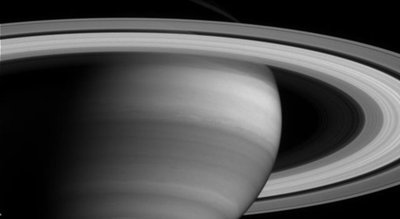The second largest planet in the solar system is Saturn. The diameter of the planet measures to about 74,898 miles. That is nine times bigger than the Earth’s diameter. A planet’s diameter is measured from the point of the atmosphere where the pressure is 1 bar. 1 bar is the pressure of Sea Level on Earth.

Saturn is the least dense of all the planets in the Solar System. Its density is only a tenth of Earth. If you were to weigh equal parts of the Earth and Saturn on the basis of Earth’s gravitational force you would find that Earth’s portion would weigh more than that of Saturn. There is no specific density of Saturn as the numbers vary. These varying numbers are fairly close to each other at, 0.69 g/cm3, 0.7 g/cm and 0.75 g/cm3.This is fairly accurate data on the true density of Saturn. At 1 g/cm water is denser than Saturn. So if you find a big enough ocean to put Saturn in, Saturn would float. Other planets that are smaller than Saturn but has a larger density, such as Neptune, would sink. Size and density are in no way connected.

Saturn may have the lowest density but Saturn’s mass is greater than all the planets except for Jupiter. Saturn’s mass is 5.688e+26. Saturn’s gravity is a little bit higher than that of Earth. It is 7/100 more than that of Earth. If you are say a hundred pounds and you suddenly visit Saturn’s surface you will immediately gain seven pounds.